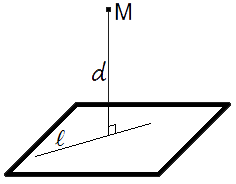Study of mathematics online.
Study math with us and make sure that "Mathematics is easy!"

# Distance from point to plane.Definition.
The distance from a point to a plane is equal to length of the perpendicular lowered from a point on a plane.

## Distance from point to plane formula

If Ax + By + Cz + D = 0 is a plane equation, then distance from point M(Mx, My, Mz) to plane can be found using the following formula:

 d = |A·Mx + B·My + C·Mz + D| √A2 + B2 + C2

## Examples of tasks with distance from point to plane

Example 1.
To find a distance between plane 2x + 4y - 4z - 6 = 0 and point M(0, 3, 6).

Solution. Let's use the formula

 d = |2·0 + 4·3 + (-4)·6 - 6| = |0 + 12 - 24 - 6| = |- 18| = 3 √4 + 16 + 16 √36 6

Answer: Distance from point to plane is equal to 3.